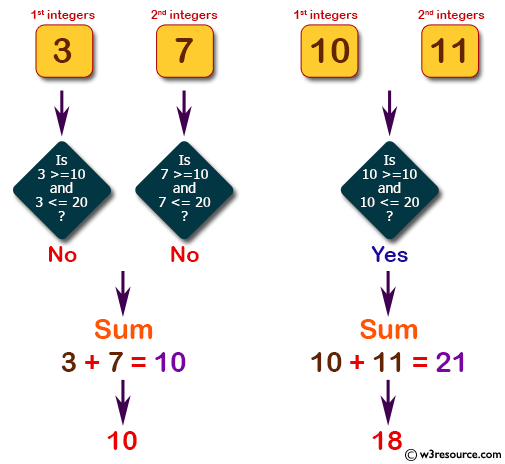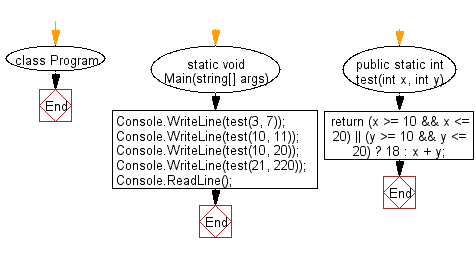﻿ C# - Compute the sum of the two given integers - w3resource# C# Sharp Basic Algorithm Exercises: Compute the sum of the two given integers

## C# Sharp Basic Algorithm: Exercise-45 with Solution

Write a C# Sharp program to compute the sum of the two given integers. If one of the given integer value is in the range 10..20 inclusive return 18.

Pictorial Presentation:Sample Solution:-

C# Sharp Code:

``````using System;
using System.Linq;
namespace exercises
{
class Program
{
static void Main(string[] args)
{
Console.WriteLine(test(3, 7));
Console.WriteLine(test(10, 11));
Console.WriteLine(test(10, 20));
Console.WriteLine(test(21, 220));

}
public static int test(int x, int y)
{
return (x >= 10 && x <= 20) || (y >= 10 && y <= 20) ? 18 : x + y;
}
}
}
```
```

Sample Output:

```10
18
18
241```

Flowchart:C# Sharp Code Editor:

Improve this sample solution and post your code through Disqus

What is the difficulty level of this exercise?

Test your Programming skills with w3resource's quiz.

﻿